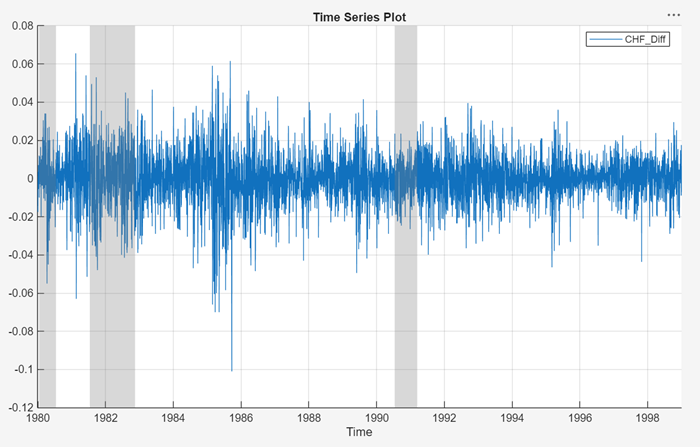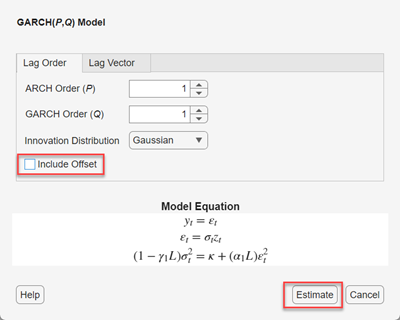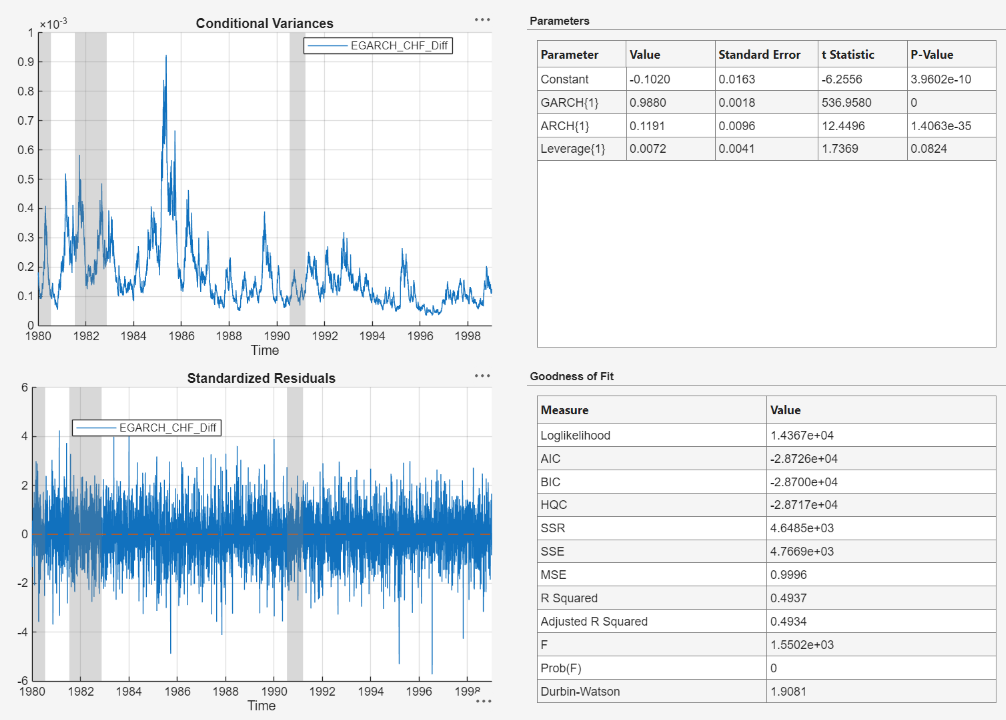## Compare Conditional Variance Model Fit Statistics Using Econometric Modeler App

This example shows how to specify and fit GARCH, EGARCH, and GJR models to data using the Econometric Modeler app. Then, the example determines the model that fits to the data the best by comparing fit statistics. The data set, which is stored in `Data_FXRates.mat`, contains foreign exchange rates measured daily from 1979–1998.

Consider creating a predictive model for the Swiss franc to US dollar exchange rate (`CHF`).

### Import Data into Econometric Modeler

At the command line, load the `Data_FXRates.mat` data set.

`load Data_FXRates`

Convert the table `DataTable` to a timetable:

1. Clear the row names of `DataTable`.

2. Convert the sampling times to a `datetime` vector.

3. Convert the table to a timetable by associating the rows with the sampling times in `dates`.

```DataTable.Properties.RowNames = {}; dates = datetime(dates,'ConvertFrom','datenum',... 'Format','ddMMMyyyy','Locale','en_US'); DataTable = table2timetable(DataTable,'RowTimes',dates);```

At the command line, open the Econometric Modeler app.

`econometricModeler`

Alternatively, open the app from the apps gallery (see Econometric Modeler).

Import `DataTable` into the app:

1. On the Econometric Modeler tab, in the Import section, click.

2. In the Import Data dialog box, in the Import? column, select the check box for the `DataTable` variable.

3. Click .

All time series variables in `DataTable` appear in the Data Browser, and a time series plot of all the series appears in the Time Series Plot(AUD) figure window.

### Plot the Series

Plot the Swiss franc exchange rates by double-clicking the `CHF` time series in the Data Browser.

Highlight periods of recession:

1. In the Time Series Plot(CHF) figure window, right-click the plot.

2. In the context menu, select Show Recessions.The `CHF` series appears to have a stochastic trend.

### Stabilize the Series

Stabilize the Swiss franc exchange rates by applying the first difference to `CHF`.

1. In the Data Browser, select `CHF`.

2. On the Econometric Modeler tab, in the Transforms section, click .

3. Highlight periods of recession:

1. In the Time Series Plot(CHFDiff) figure window, right-click the plot.

2. In the context menu, select Show Recessions.

A variable named `CHFDiff`, representing the differenced series, appears in the Data Browser, and its time series plot appears in the Time Series Plot(CHFDiff) figure window.The series appears to be stable, but it exhibits volatility clustering.

### Assess Presence of Conditional Heteroscedasticity

Test the stable Swiss franc exchange rate series for conditional heteroscedasticity by conducting Engle's ARCH test. Run the test assuming an ARCH(1) alternative model, then run the test again assuming an ARCH(2) alternative model. Maintain an overall significance level of 0.05 by decreasing the significance level of each test to 0.05/2 = 0.025.

1. In the Data Browser, select `CHFDiff`.

2. On the Econometric Modeler tab, in the Tests section, click > Engle's ARCH Test.

3. On the ARCH tab, in the Parameters section, set Number of Lags of `1`.

4. Set Significance Level to `0.025`.

5. In the Tests section, click .

6. Repeat steps 3 through 5, but set Number of Lags to `2` instead.

The test results appear in the Results table of the ARCH(CHFDiff) document.The tests reject the null hypothesis of no ARCH effects against the alternative models. This result suggests specifying a conditional variance model for `CHFDiff` containing at least two ARCH lags. Conditional variance models with two ARCH lags are locally equivalent to models with one ARCH and one GARCH lag. Consider GARCH(1,1), EGARCH(1,1), and GJR(1,1) models for `CHFDiff`.

### Estimate GARCH Model

Specify a GARCH(1,1) model and fit it to the `CHFDiff` series.

1. In the Data Browser, select the `CHFDiff` time series.

2. Click the Econometric Modeler tab. Then, in the Models section, click the arrow to display the models gallery.

3. In the models gallery, in the GARCH Models section, click GARCH.

4. In the GARCH Model Parameters dialog box, on the Lag Order tab:

1. Set GARCH Degree to `1`.

2. Set ARCH Degree to `1`.3. Click .

The model variable GARCH_CHFDiff appears in the Models section of the Data Browser, and its estimation summary appears in the Model Summary(GARCH_CHFDiff) document.### Specify and Estimate EGARCH Model

Specify an EGARCH(1,1) model containing a leverage term at the first lag, and fit the model to the `CHFDiff` series.

1. In the Data Browser, select the `CHFDiff` time series.

2. On the Econometric Modeler tab, in the Models section, click the arrow to display the models gallery.

3. In the models gallery, in the GARCH Models section, click EGARCH.

4. In the EGARCH Model Parameters dialog box, on the Lag Order tab:

1. Set GARCH Degree to `1`.

2. Set ARCH Degree to `1`. Consequently, the app includes a corresponding leverage lag. You can remove or adjust leverage lags on the Lag Vector tab.

5. Click .

The model variable EGARCH_CHFDiff appears in the Models section of the Data Browser, and its estimation summary appears in the Model Summary(EGARCH_CHFDiff) document.### Specify and Estimate GJR Model

Specify a GJR(1,1) model containing a leverage term at the first lag, and fit the model to the `CHFDiff` series.

1. In the Data Browser, select the `CHFDiff` time series.

2. On the Econometric Modeler tab, in the Models section, click the arrow to display the models gallery.

3. In the models gallery, in the GARCH Models section, click GJR.

4. In the GJR Model Parameters dialog box, on the Lag Order tab:

1. Set GARCH Degree to `1`.

2. Set ARCH Degree to `1`. Consequently, the app includes a corresponding leverage lag. You can remove or adjust leverage lags on the Lag Vector tab.

3. Click .

The model variable GJR_CHFDiff appears in the Models section of the Data Browser, and its estimation summary appears in the Model Summary(GJR_CHFDiff) document.### Choose Model

Choose the model with the best parsimonious in-sample fit. Base your decision on the model yielding the minimal Bayesian information criterion (BIC). The table shows the BIC fit statistics of the estimated models, as given in the Goodness of Fit section of the estimation summary of each model.

ModelBIC
GARCH(1,1)-28707
EGARCH(1,1)-28700
GJR(1,1)-28711

The GJR(1,1) model yields the minimal BIC value. Therefore, it has the best parsimonious in-sample fit of all the estimated models.